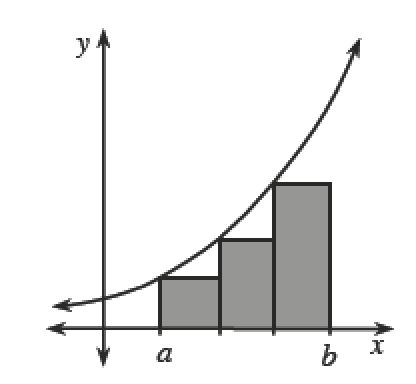### Home > APCALC > Chapter 8 > Lesson 8.1.1 > Problem8-4

8-4.

Remember that $\lim\limits _ { n \rightarrow \infty } \displaystyle\sum _ { i = 0 } ^ { n - 1 } f ( a + ( \Delta x ) i ) \Delta x = \int _ { a } ^ { b } f ( x ) d x$.

1. What does "$dx$" by itself represent?

What is the connection between $dx$ and $Δx$?

2. What does the product $f(x)dx$ represent?

What is the connection between $f(x)dx$ and $f(a + Δxi)Δx$?